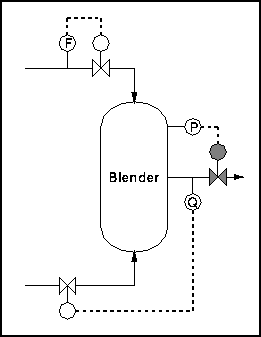# 2.2: Degrees of Freedom- importance, calculation procedure, and examples

•• Contributed by Peter Woolf et al.
• Assistant Professor (Chemical Engineering) at University of Michigan

Authors: (13 December 2009) Jon Butler, Matthew J LaVelle

## 2.1 Introduction

In control engineering, a degree of freedom analysis is necessary to determine the regulatable variables within the chemical process. These variables include descriptions of state such as pressure or temperature as well as compositions and flow rates of streams.

## 2.2 Definition

The number of process variables over which the operator or designer may exert control. Specifically, control degrees of freedom include:

1. The number of process variables that may be manipulated once design specifications are set
2. The number of said manipulated variables used in control loops
3. The number of single-input, single-output control loops
4. The number of regulated variables contained in control loops

The following procedure identifies potential variables for manipulation.

## 2.3 The Process

The method we will discuss is the Kwauk method, developed by Kwauk and refined by Smith. The general equation follows:

Degrees of freedom = unknowns - equations

Unknowns are associated with mass or energy streams and include pressure, temperature, or composition. If a unit had Ni inlet streams, No outlets, and C components, then for design degrees of freedom, C+2 unknowns can be associated with each stream. This means that the designer would be manipulating the temperature, pressure, and stream composition.

This sums to an equation of

Total Unknowns = Ni*(C+2) + No*(C+2)

If the process involves an energy stream there is one unknown associated with it, which is added to this value.

Equations may be of several different types, including mass or energy balances and equations of state such as the Ideal Gas Law.

After Degrees of Freedom are determined, the operator assigns controls. Carrying out a DOF analysis allows planning and understanding of the chemical process and is useful in systems design.

## 2.4 Applications

Single phase systems

• All outlet streams have the same composition, and can be assumed to have the same temperature and pressure

Multiple phase systems

• An additional (C-1) composition variable exists for each phase

Complete Process

• When connecting units which share streams, one degree of freedom is lost from the total of the individual units

## 2.5 Example 1: Blender

This example (from the ECOSSE Control Course web page listed below) investigates degrees of freedom in a simple vapor mixing unit. Two gaseous streams enter a vessel and exit as a single well-mixed stream (Figure 1). We will apply the above equation to determine degrees of freedom.Figure 1: Blender Schematic

Here, there are 3 streams, each with C+2 unknowns for a total of 3C+6 Unknowns.

We have C mass balances and 1 energy balance for a total of 2C+1 equations. We also know composition, pressure, and temperature for the incoming streams. This adds 2C+2 to the equation. Putting everything together gives:

Degrees of freedom = 3C+6 - (2C+1 + 2C+2)

Hence, the system has 3 degrees of freedom. Therefore, we can fix outlet composition, pressure, and flow rate. Figure 2 shows an example control scheme:Figure 2: Blender with controls

## 2.6 References

Ponton JW, 1994, Degrees of Freedom Analysis in Process Control, Chemical Engineering Science, Vol. 49, No. 13, pp 1089 - 1095.

eweb.chemeng.ed.ac.uk/courses/control/restricted/course/third/course/formal.html